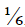×
Get Full Access to Probability And Statistical Inference - 9 Edition - Chapter 1.1 - Problem 5e
Get Full Access to Probability And Statistical Inference - 9 Edition - Chapter 1.1 - Problem 5e

×

# Consider the trial on which a 3 is first observed inISBN: 9780321923271 41

## Solution for problem 5E Chapter 1.1

Probability and Statistical Inference | 9th Edition

• Textbook Solutions
• 2901 Step-by-step solutions solved by professors and subject experts
• Get 24/7 help from StudySoup virtual teaching assistantsProbability and Statistical Inference | 9th Edition

4 5 1 236 Reviews
28
0
Problem 5E

Consider the trial on which a 3 is first observed in successive rolls of a six-sided die. Let A be the event that 3 is observed on the first trial. Let B be the event that at least two trials are required to observe a 3. Assuming that each side has probability 1/6, find (a) P(A), (b) P(B), and (c) P(A B).

Step-by-Step Solution:

Step 1 of 4

We have the trial on which a 3 is first observed in successive rolls of a six-sided die.

Let A be the event that 3 is observed on the first trial.

Let B be the event that at least two trials are required to observe a 3. And

probability of each side has.

We need to find,

(a) P(A),

(b) P(B), and

(c) P(AB).

Step 2 of 4

Step 3 of 4

##### ISBN: 9780321923271

Unlock Textbook Solution